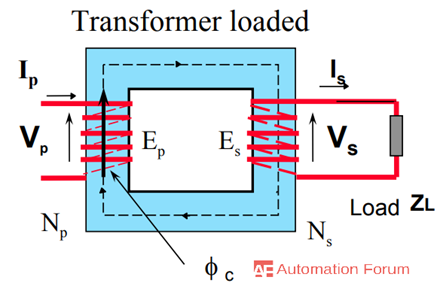# What is real and ideal transformer and how are they different from each other

What is a transformer

A transformer is a device that converts one AC voltage to another AC voltage without changing the frequency. It consists of one or more coils of wire wrapped around a common ferromagnetic core and these coils are not electrically connected, but they are connected through the common magnetic flux confined to the core. A transformer can be considered as a stationary electric machine which transfers electrical energy from one voltage to another, the transformer is a static device and all currents and voltages are AC. The transfer of energy takes place through the magnetic field.

What is an ideal transformer

An ideal transformer can be considered as a transformer without any energy loss, these transformers are linear. In an ideal transformer, they have perfect coupling, which would create high core magnetic permeability and winding inductance. Ideal transformers have lossless winding, it can be defined as a unity coupled, in which the primary and secondary coils have infinite self-inductances. The windings of an ideal transformer have no ohmic resistance because of that there is no I2 R and core loss. All these features can be considered theoretically for a transformer, but practically these features won’t be present in a real transformer.If the transformer is ideal then input power = output power, if the power factor is the same on both sides then Vp Ip = Vs Is

What are the conditions of an ideal transformer

An ideal transformer won’t have any losses, its core is infinitely permeable and all fluxes link all coils, and there are no leakage fluxes. Induced voltages equal applied voltages, so the windings have no resistance. The reluctance of the core is zero and negligible current is required to establish magnetic flux, so the core has infinite permeability. There are no hysteresis or eddy currents, so they have a loss-less magnetic core. These are the conditions required for an ideal transformer.

What are the features of an ideal transformer

It would be easier to understand the operation of a transformer if we consider the transformer as an ideal transformer. In order to consider a transformer as an ideal transformer, it must have the following features, the windings must have perfect continuity, so the copper losses in either the secondary or primary coil is zero. The energy dissipation from hysteresis and eddy currents in the magnetic core is zero. The magnetic permeability of the core is perfect and constant, reluctance or magnetic resistance is zero, so the leakage inductance is zero. There is no need for magnetizing current, in order to set up the magnetic flux. Due to constant permeability, the core shows linear magnetic characteristics. Because of all these features, the ideal transformer is a no-loss device which can be able to transform even DC voltages.

How ideal transformer is different from a real transformer

In an ideal transformer, there would be no loss and the core is definitely permeable. The flux produced by the primary is completely linked by the secondary and vice versa, and there would be no leakage flux of any kind. In a real transformer, not all the flux produced by the primary current link the winding, but there is leakage of some flux into the air surrounding the primary and vice versa. There would be no loss in an ideal transformer, whereas in the case of a real transformer its coil has inductance, capacitance, and resistance, so there will be losses like winding resistance, copper loss, and eddy current.

What is a real or practical transformer

It is not possible to obtain an ideal transformer practically, but one which is closer to it can be built and it can be called the real transformer. In an ideal transformer, there is no loss, but in real transformer, there is a loss. There is three major loss in a real transformer they are core loss, copper loss, and leakage flux. Core loss is the loss by the hysteresis and eddy current loss, it is also known as iron loss. Copper loss is the loss that occurs by resistive heating and the loss will be through the primary and secondary windings. There will be no power loss due to the leakage reactance, but the presence of leakage reactance in the windings changes the power factor.

Transformer polarity

Transformer polarity can be determined by the dot convention, the dots help to determine the polarity of voltage and current in the core without physically examine the windings. A current flowing into the dotted end of the winding produces a positive magnetomotive force, while the current flowing into the un-dotted end of a winding produces a negative magnetomotive force.

Transformer voltage regulation

Voltage regulation of a transformer is defined as the variation of secondary voltage between no load and full load with the primary voltage being assumed as constant. It can be expressed as either per unit or a percentage of no-load voltage, this is also known as regulation down.